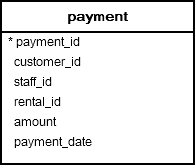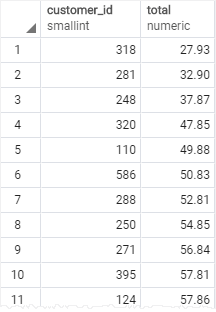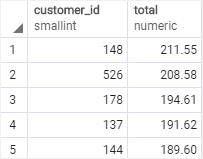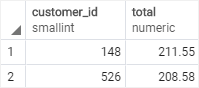# PostgreSQL Tutorial: SUM Function

August 3, 2023

Summary: in this tutorial, you’ll learn how to use PostgreSQL `SUM()` function to calculate the sum of a set of values.

## Introduction to PostgreSQL SUM() function

The PostgreSQL `SUM()` is an aggregate function that returns the sum of values or distinct values.

The syntax of the `SUM`() function is as follows:

``````SUM(DISTINCT expression)
``````

The `SUM()` function ignores `NULL`. It means that `SUM()` doesn’t consider the `NULL` in calculation.

If you use the `DISTINCT` option, the `SUM()` function calculates the sum of distinct values.

For example, without the `DISTINCT` option, the `SUM()` of 1, 1, 8, and 2 will return 12. When the `DISTINCT` option is available, the `SUM()` of 1, 1, 8, and 2 will return 11 (1 + 8 + 2). It ingores the one duplicate value (1).

If you use the `SUM` function in a `SELECT` statement, it returns `NULL` not zero in case the `SELECT` statement returns no rows.

## PostgreSQL SUM() function examples

We’ll use the `payment` table in the sample database to demonstrate the functionality of the `SUM` function.### 1) Using PostgreSQL SUM() function in SELECT statement example

The following statement uses the `SUM()` function to calculate the total payment of the customer id 2000.

``````SELECT SUM (amount) AS total
FROM payment
WHERE customer_id = 2000;
``````
`````` total
-------
null
(1 row)
``````

Since no row in the `payment` table has the `customer_id` 2000, the `SUM()` function returns a `NULL`.

If you want the `SUM()` function to return zero instead of `NULL` in case there is no matching row found, you use the `COALESCE` function.

The `COALESCE()` function returns the first non-null argument. In other words, it returns the second argument if the first argument is `NULL`.

The following query illustrates how to use the `SUM()` function with the `COALESCE()` function:

``````SELECT
COALESCE(SUM(amount),0) AS total
FROM
payment
WHERE
customer_id = 2000;
``````
`````` total
-------
0
(1 row)
``````

### 2) Using PostgreSQL SUM() function with GROUP BY clause

To calculate the summary of every group, you use the `GROUP BY` clause to group the rows in the table into groups and apply the `SUM()` function to each group.

The following example uses the `SUM()` function with the `GROUP BY` clause to calculate the total amount paid by each customer:

``````SELECT
customer_id,
SUM (amount) AS total
FROM
payment
GROUP BY
customer_id
ORDER BY total;
``````The following query returns top five customers who paid the most:

``````SELECT
customer_id,
SUM (amount) AS total
FROM
payment
GROUP BY
customer_id
ORDER BY total DESC
LIMIT 5;
``````### 3) Using PostgreSQL SUM function with HAVING clause

To filter the sums of groups based on a specific condition, you use the `SUM` function in the `HAVING` clause.

The following example returns the customers who paid more than \$200:

``````SELECT
customer_id,
SUM (amount) AS total
FROM
payment
GROUP BY
customer_id
HAVING SUM(amount) > 200
ORDER BY total DESC
``````### 4) Using PostgreSQL SUM with expression

See the following `rental` table from the sample database:The following statement uses the `SUM()` function to calculate total rental days:

``````SELECT SUM(return_date - rental_date )
FROM rental;
``````
``````           sum
-------------------------
71786 days 190098:21:00
(1 row)
``````

How it works.

• First, calculate the rental duration by subtracting the rental date from the return date.
• Second, apply the `SUM()` function to the expression.

The following example uses the `SUM()` function to calculate the total duration by customers:

``````SELECT first_name || ' ' || last_name full_name,
SUM(return_date - rental_date ) rental_duration
FROM rental
INNER JOIN customer USING(customer_id)
GROUP BY customer_id
ORDER BY full_name;
``````## Summary

• Use the `SUM()` function to calculate the sum of values.
• Use the `DISTINCT` option to calculate the sum of distinct values.
• Use the `SUM()` function with the `GROUP BY` clause to calculate the sum for each group.